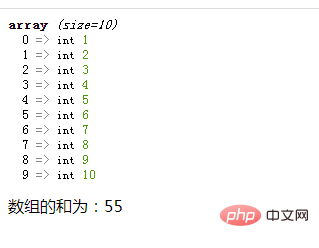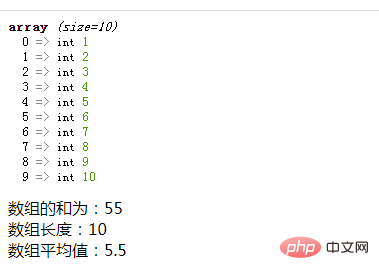# php数组求和与平均值的函数是什么

php中有数组求和函数“array_sum()”，可以计算一维数组中所有元素的和；但没有直接求数组平均值的函数。php数组想要求平均值，可以利用array_sum()、count()函数与“/”运算符实现，方法：1、使用“array_sum(\$arr)”语句计算出数组元素和；2、使用“count(\$arr)”语句计算出数组长度；3、用“数组元素和/数组长度”语句求出平均值即可。php中提供了数组求和函数“array_sum()”，但没有提供直接求数组平均值的函数。

• 利用`array_sum(\$arr)`可以计算一维数组中所有元素的和

```<?php
\$arr = array(1,2,3,4,5,6,7,8,9,10);
var_dump(\$arr);
\$sum=array_sum(\$arr);
echo "数组的和为：".\$sum;
?>```• 使用array_sum()计算出数组元素和；

• 使用count()计算出数组长度；

• 使用“数组元素和/数组长度”语句求出平均值

```<?php
\$arr = array(1,2,3,4,5,6,7,8,9,10);
var_dump(\$arr);
\$sum=array_sum(\$arr);
echo "数组的和为：".\$sum."<br>";
\$len=count(\$arr);
echo "数组长度：".\$len."<br>";
\$average=\$sum/\$len;
echo "数组平均值：".\$average."<br>";
?>```1/1